Function Repository Resource:

# SternBrocotTree

Generate a complete binary search tree of positive fractions

Contributed by: Ian Ford (Wolfram Research)
 ResourceFunction["SternBrocotTree"][n] generates a complete binary search tree of the positive fractions down to level n.

## Details and Options

The fractions on level d are those for which the total of the terms in its continued fraction representation is d+1.
In ResourceFunction["SternBrocotTree"][n], n can be any non-negative machine integer.
ResourceFunction["SternBrocotTree"] takes the same options as Tree.

## Examples

### Basic Examples (1)

Generate a complete binary search tree of the positive fractions down to level 4:

 In:=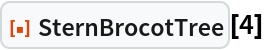Out=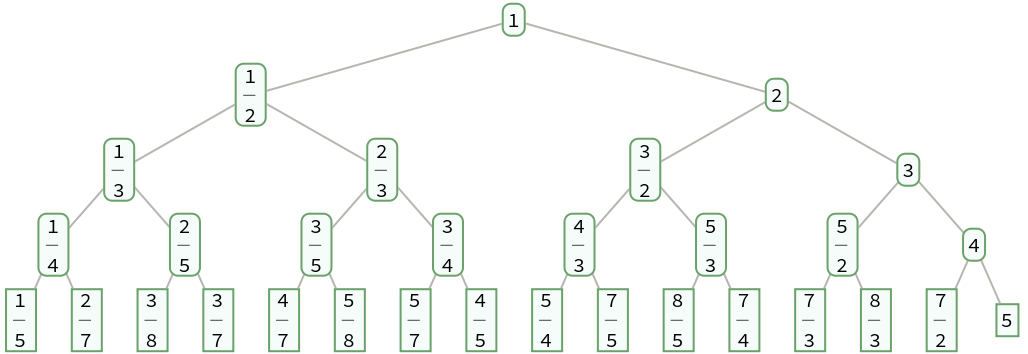### Applications (1)

Approximate Pi as a rational number with a continued fraction representation with terms totalling 10:

 In:=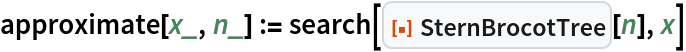In:=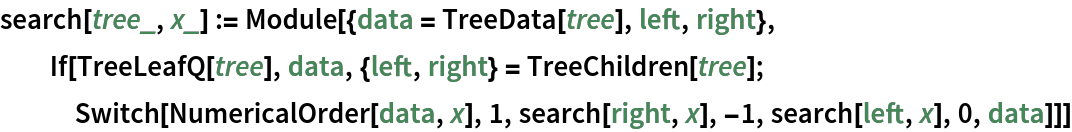In:=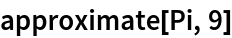Out=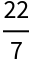In:=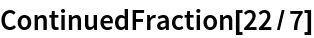Out=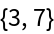### Properties and Relations (7)

gives the list of fractions in SternBrocotTree[n-1] between 0 and 1 with denominators not exceeding n:

 In:=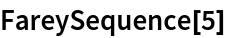Out=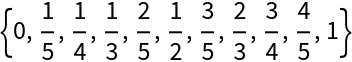In:=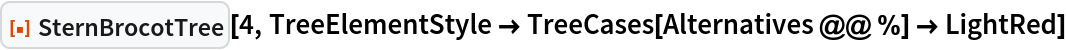Out=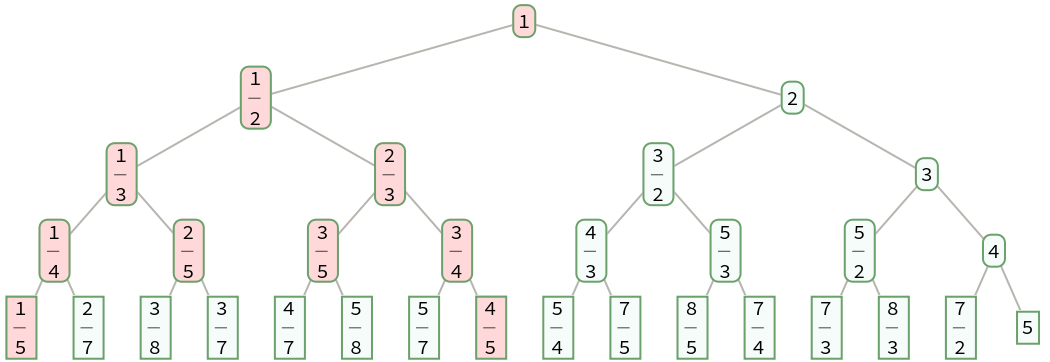SternBrocotTree gives a sorted binary tree, also known as a search tree:

 In:=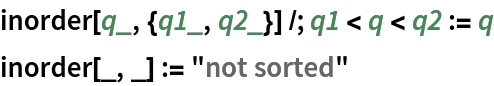In:=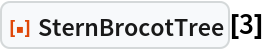Out=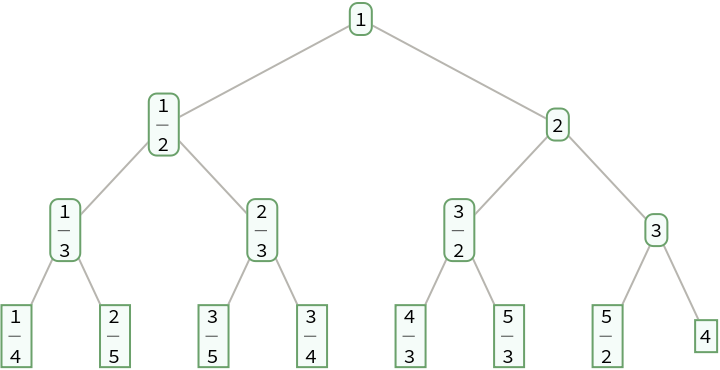In:=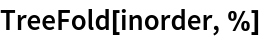Out=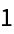A fraction with a continued fraction representation {a1,,ak} has children with continued fraction representations {a1,,ak-1,2} and {a1,,ak+1}:

 In:=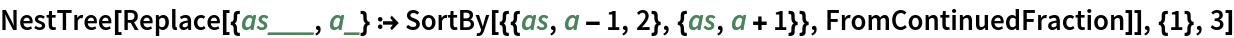Out=In:=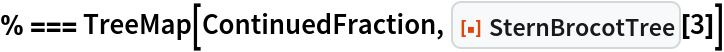Out=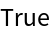The fractions on level d are those for which the total of the terms in its continued fraction representation is d+1:

 In:=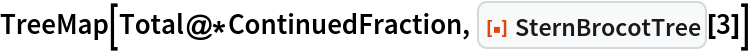Out=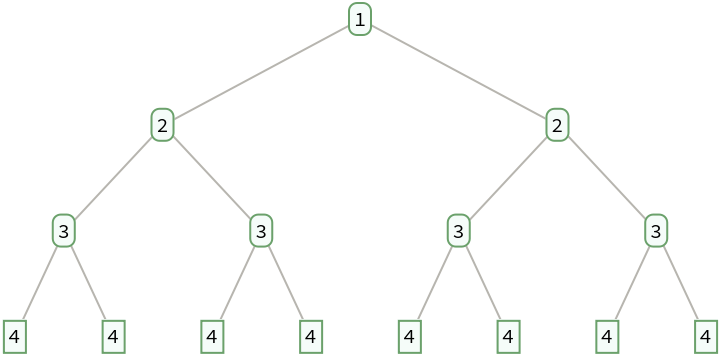The fractions given by SternBrocotTree can be obtained by successively taking the sums of the numerators and denominators of consecutive pairs of fractions starting with 0 and Infinity:

 In:=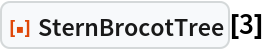Out=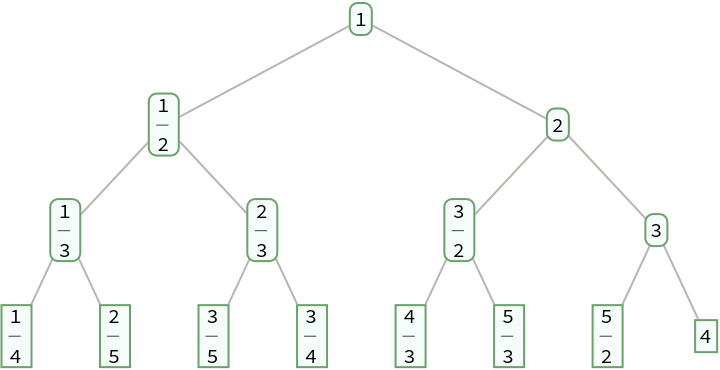In:=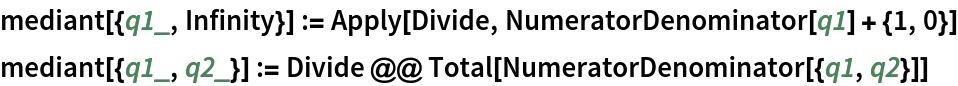In:=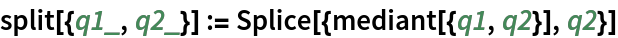In:=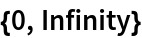Out=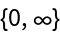In:=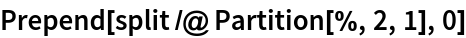Out=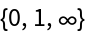In:=Out=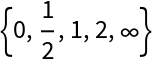In:=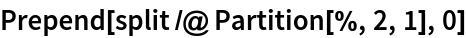Out=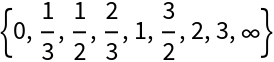In:=Out=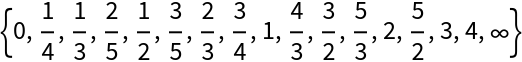The convergents of a positive fraction are a subset of its ancestors in the Stern-Brocot tree:

 In:=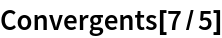Out=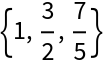In:=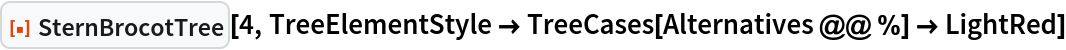Out=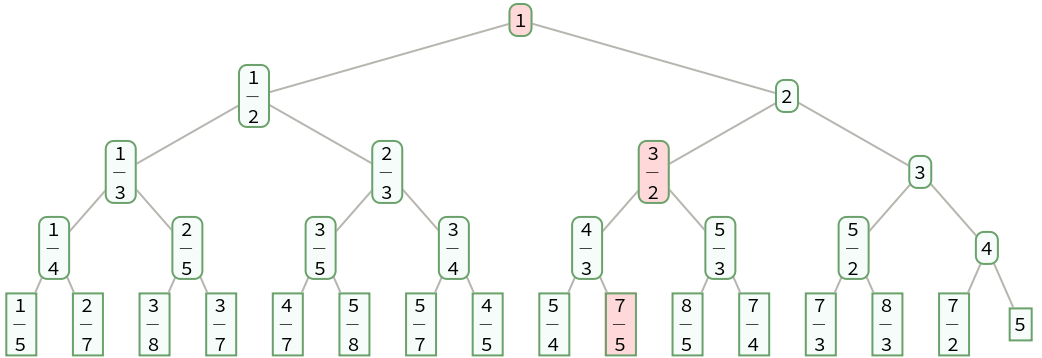In:=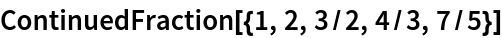Out=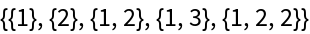If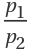,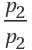, ,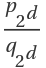are the fractions at level d, then:

 In:=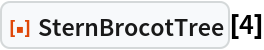Out=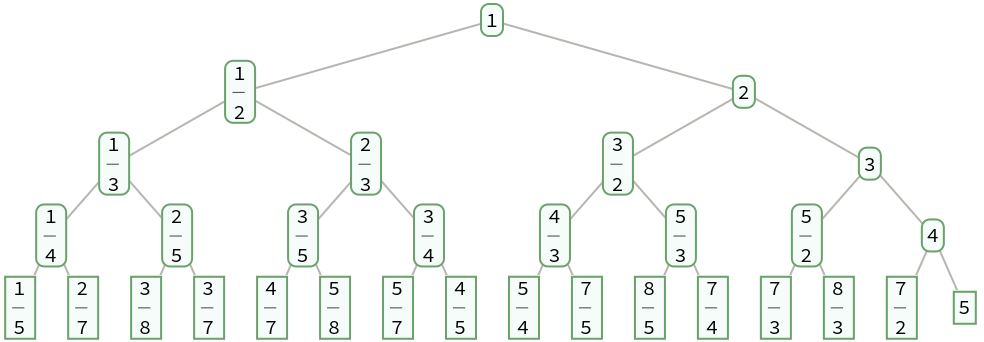In:=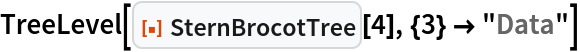Out=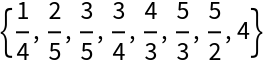In:=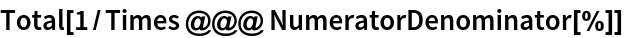Out=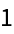Define a function that permutes the positions on level d by reversing the corresponding binary integers with d digits:

 In:=Permute the fractions on each level of a Stern-Brocot tree:

 In:=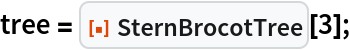In:=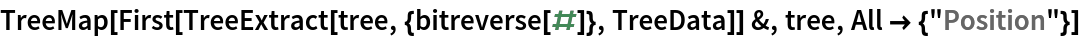Out=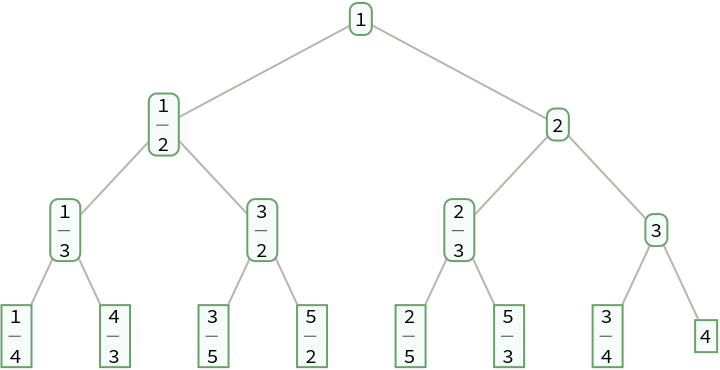The result is a Calkin-Wilf tree:

 In:=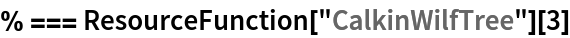Out=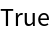## Version History

• 1.0.0 – 06 January 2023